Filters
Size

# Full Price Tees

Filters

Selected Filters• XS
• S
• M
• L
• XL
• XXL
• Select Size
Sold Out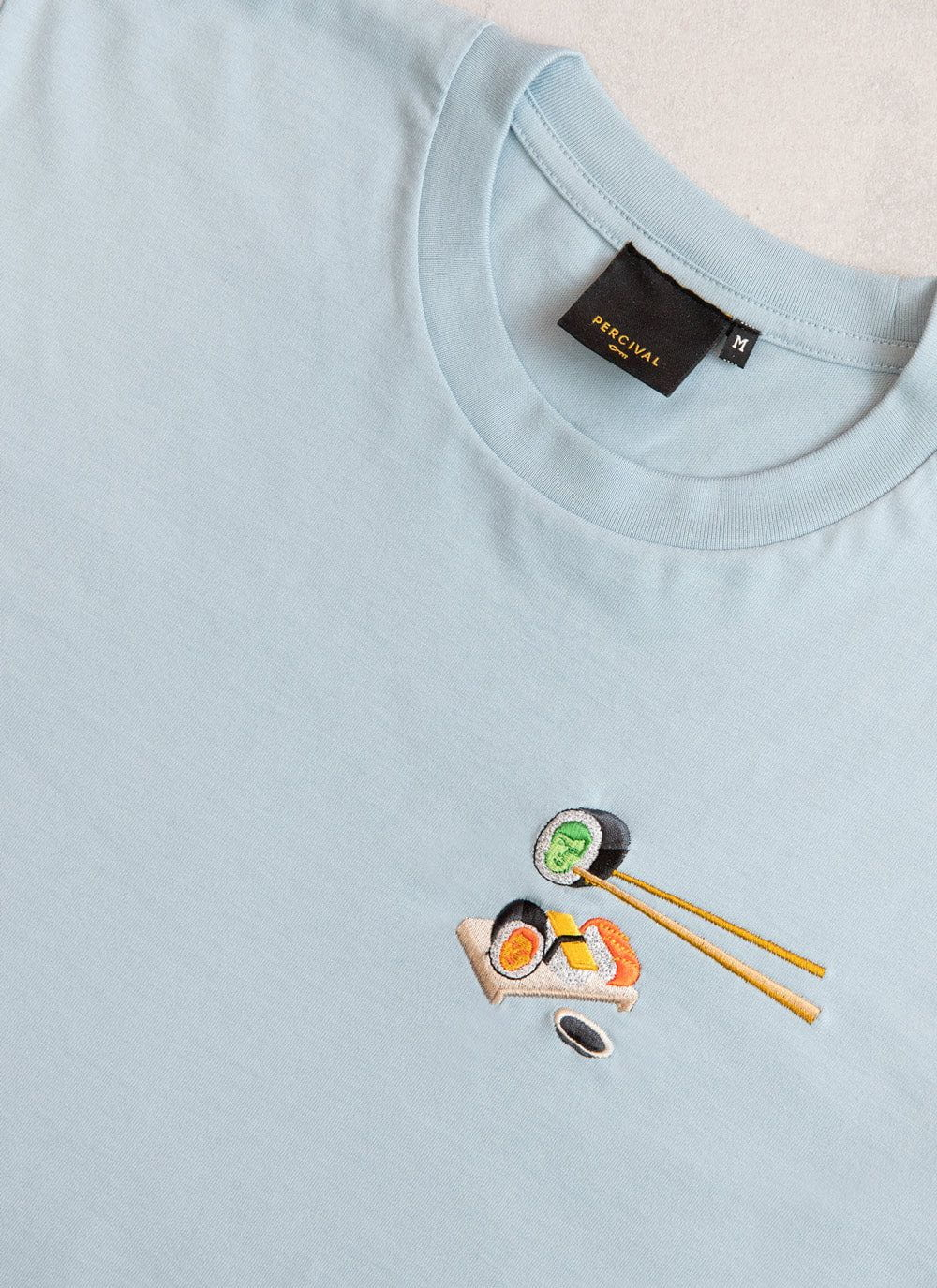• XS
• S
• M
• L
• XL
• XXL
• Select Size
Sold Out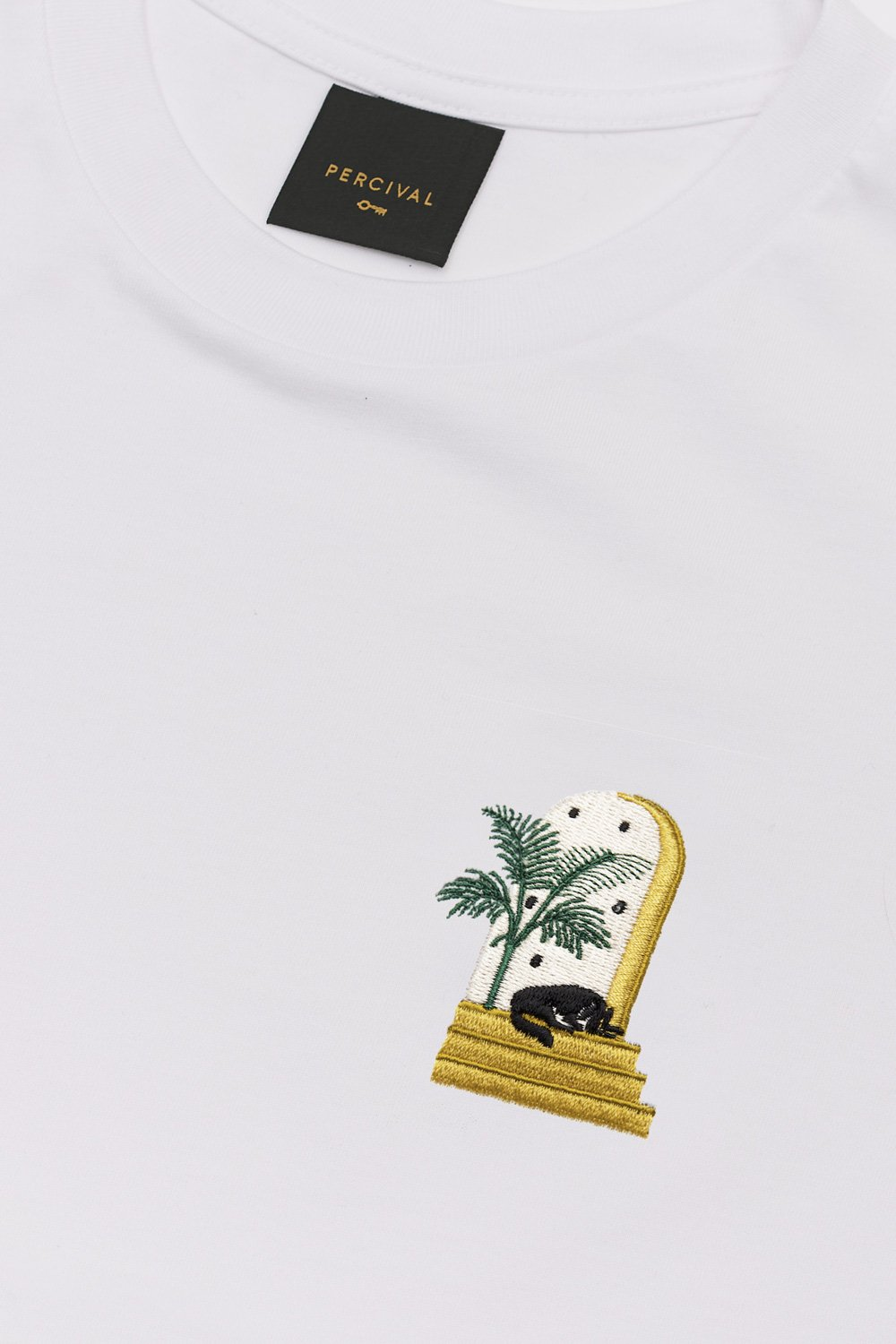• XS
• S
• M
• L
• XL
• XXL
• Select Size• XS
• S
• M
• L
• XL
• XXL
• Select Size• XS
• S
• M
• L
• XL
• XXL
• Select Size• XS
• S
• M
• L
• XL
• XXL
• Select Size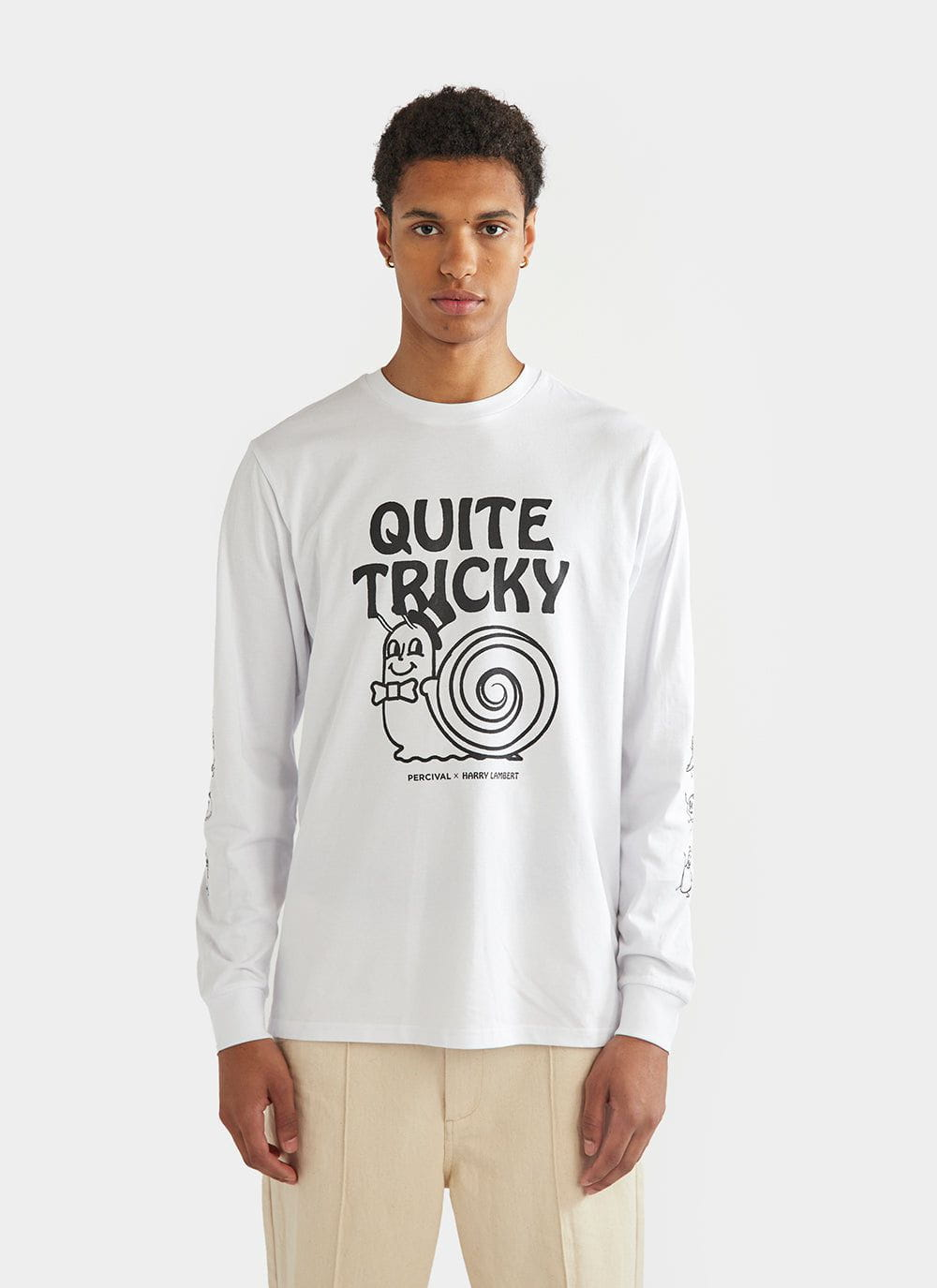• XS
• S
• M
• L
• XL
• XXL
• Select Size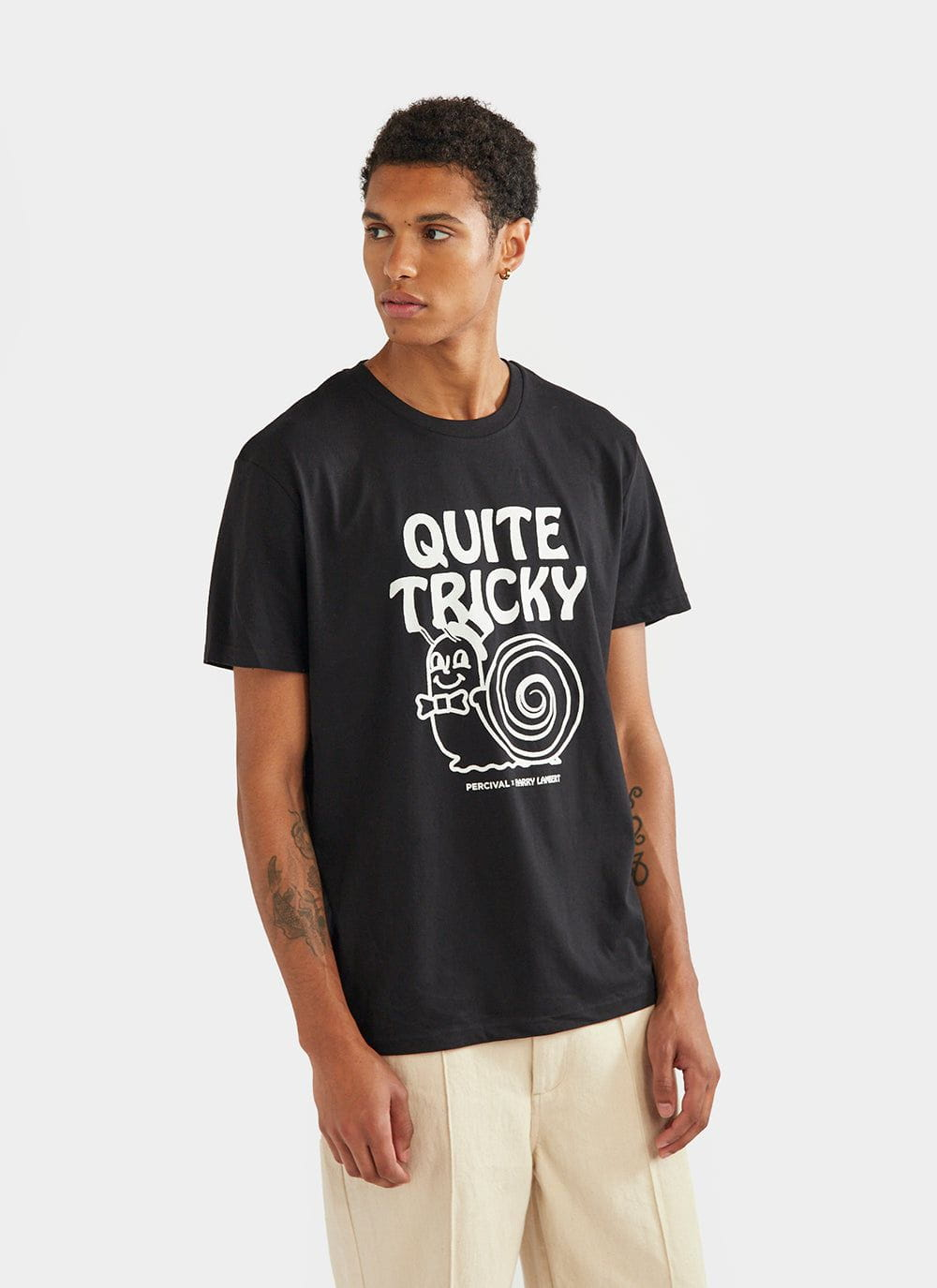• XS
• S
• M
• L
• XL
• XXL
• Select Size• XS
• S
• M
• L
• XL
• XXL
• Select Size
New Arrival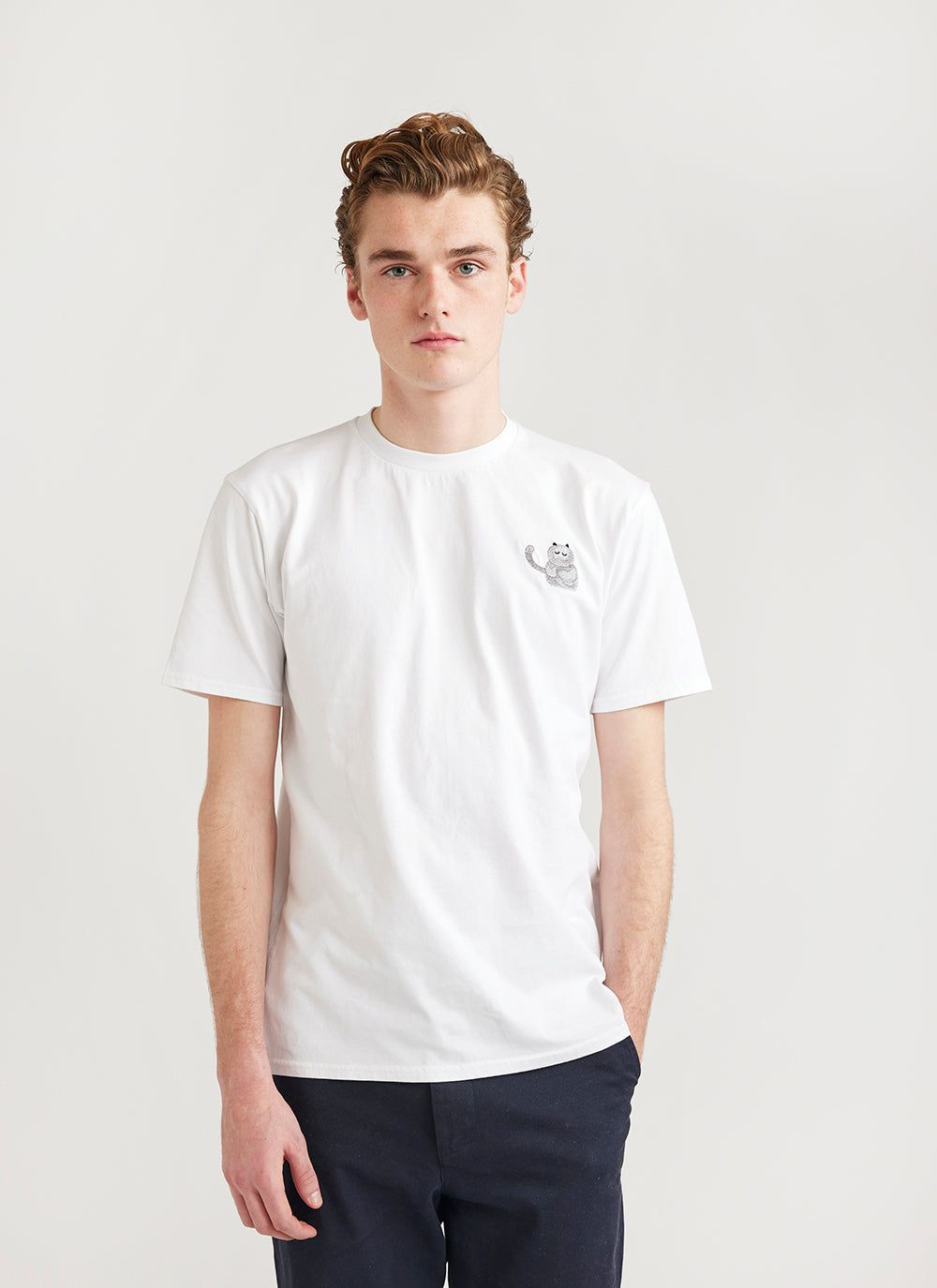• XS
• S
• M
• L
• XL
• XXL
• Select Size
Running Low New Arrival• XS
• S
• M
• L
• XL
• XXL
• Select Size
Sold Out New Arrival• XS
• S
• M
• L
• XL
• XXL
• Select Size